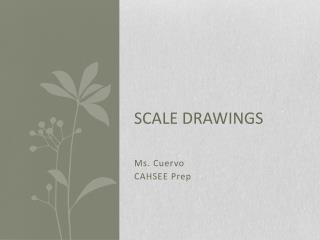DownloadDownload PresentationScale Drawings

# Scale Drawings

Télécharger la présentation## Scale Drawings

- - - - - - - - - - - - - - - - - - - - - - - - - - - E N D - - - - - - - - - - - - - - - - - - - - - - - - - - -
##### Presentation Transcript

1. Scale Drawings Ms. Cuervo CAHSEE Prep

2. What Are Scale Drawings? Since it is not always possible to draw on paper the actual size of real-life objects such as the real size of a car, an airplane, we need scale drawings to represent the size like the one you see below of a van. Notice that the first number always refers to the length of the drawing on paper and the second number refers to the length of real-life object In real-life, the length of this van may measure 240 inches. However, the length of a copy or print paper that you could use to draw this van is a little bit less than 12 inches

3. Examples of Scale Drawings and How They’re Used

4. Examples of Scale Drawings

5. Scale Drawings & Maps

6. Opening Activity You and a friend are following a map to drive to Tijuana. Your friend notices that the key on the map says “1 inch = 50 miles.” Why do you think the creator of this map did not choose to represent their actual size? Explain what “1 inch = 5o miles” means.

7. Introduction All maps are reductions of a physical space. The distance you see on a map is much smaller than the actual distance it represents. Map makers include a scale to tell the user how much they have reduced the distance. The scale allows you to measure a distance on a map and calculate the actual distance .

8. Length = 5 inches Question: You are given the scale drawing below of a room in house. If the actual width is 7 feet, what is the actual length of the room? Width = 2 in. Step One: What is asked? Underline the question. What is the actual length of the room? Step Two: What information is given? Highlight the information. Actual width = 7 feet Step Three: Is a ratio given? If so, set up the problem.

9. Question: You are given the scale drawing below of a room in house. If the actual width is 7 feet, what is the actual length of the room? Length = 5 inches Begin setting up your equation. On the right side of the equation, write the conversion between inches on the drawing and actual feet. On the left side of the equation, write the remaining measurement and the unknown value, keeping the same units in the numerator and denominator. Cross-multiply and solve for the unknown value. Width = 2 in.

10. Length= ? Length = 5 in Width= 7 feet Fake 5 in = 2 in Real x feet 7 feet 2x = 35 x = 17.5 feet Width = 2 in